# Notes on RFDS 2

``library(tidyverse)``
``````## Loading tidyverse: ggplot2
``## Conflicts with tidy packages ----------------------------------------------``
``````## filter(): dplyr, stats
## lag():    dplyr, stats``````

## Facets

Bill Cleveland called these graphics trellises and implemented them in the original S language in the package Trellis.

Trellis was essentially re-created in R under the name lattice by Deepayan Sarkar. See http://lmdvr.r-forge.r-project.org/figures/figures.html. See figures 2.8 - 2.11 on Titanic survivors.

Trellis and Lattice are strongly focused on this type of graphic and have declined in popularity since the grammar of graphics aproach ggplot2.

Tufte refers to this type of graphic as ‘small multiples.’

## facet_wrap

To produce separate graphs for each value of a single categorical variable use facet_wrap() as a layer. The syntax requires a single-variable formula in a formula (preceded by a ~).

Example

``````d = ggplot(data=mpg, aes(x=cty,y=hwy)) + geom_point()

d + facet_wrap(~class)``````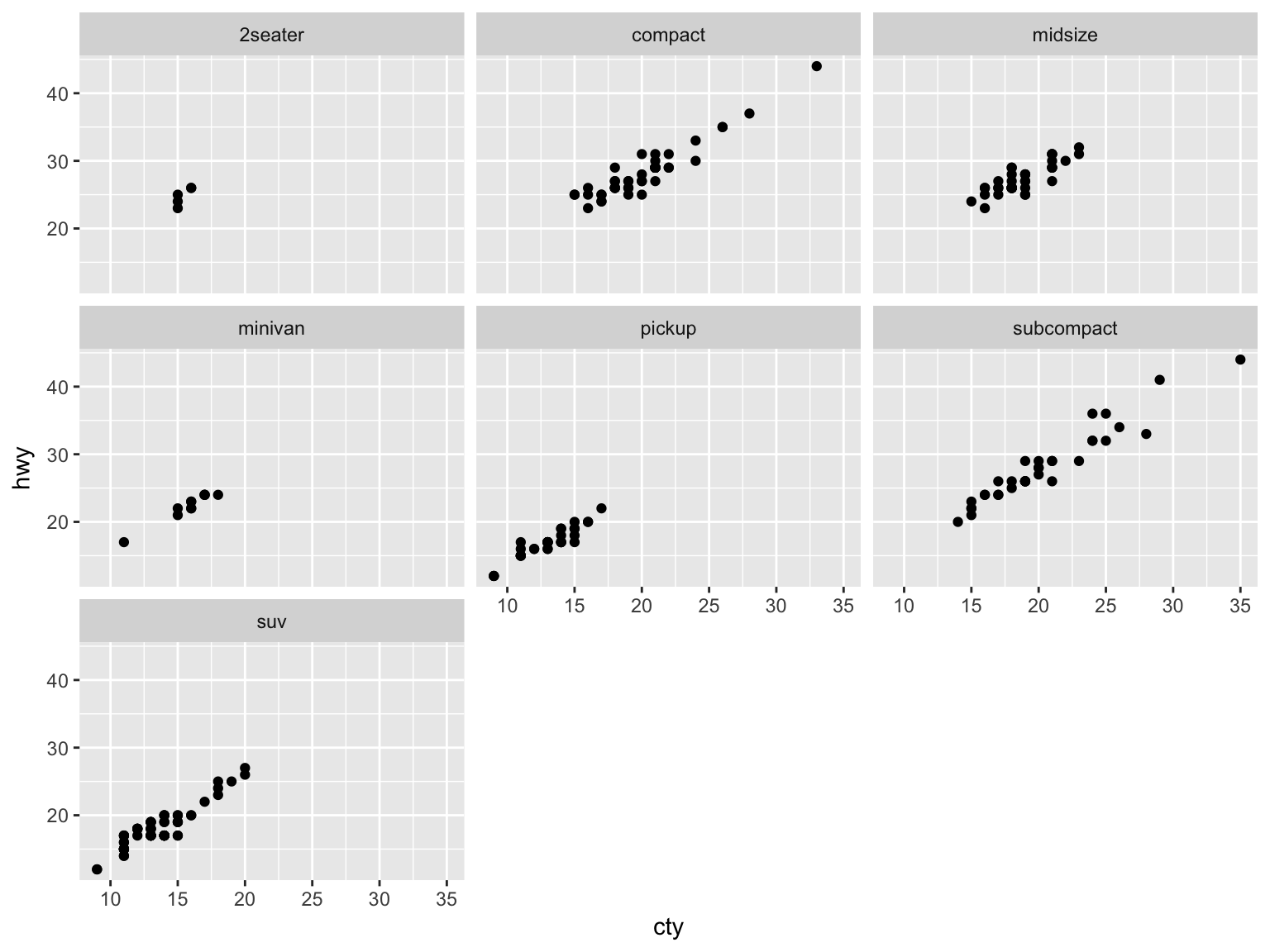The layout can be controlled with the arguments ncol or nrow.

``d + facet_wrap(~class,nrow=2) + ggtitle("nrow = 2")``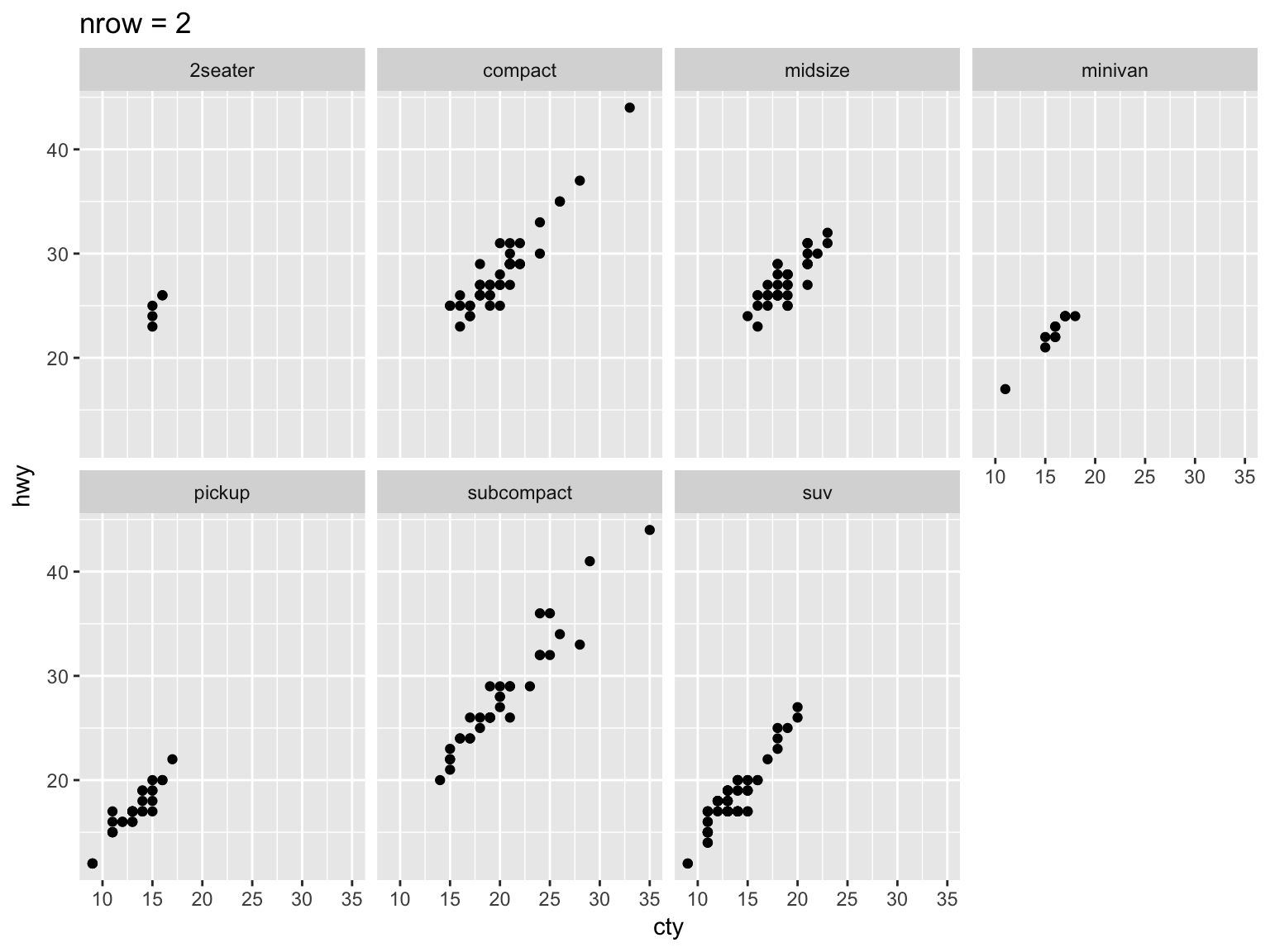``d + facet_wrap(~class,ncol=2) + ggtitle("ncol=2")``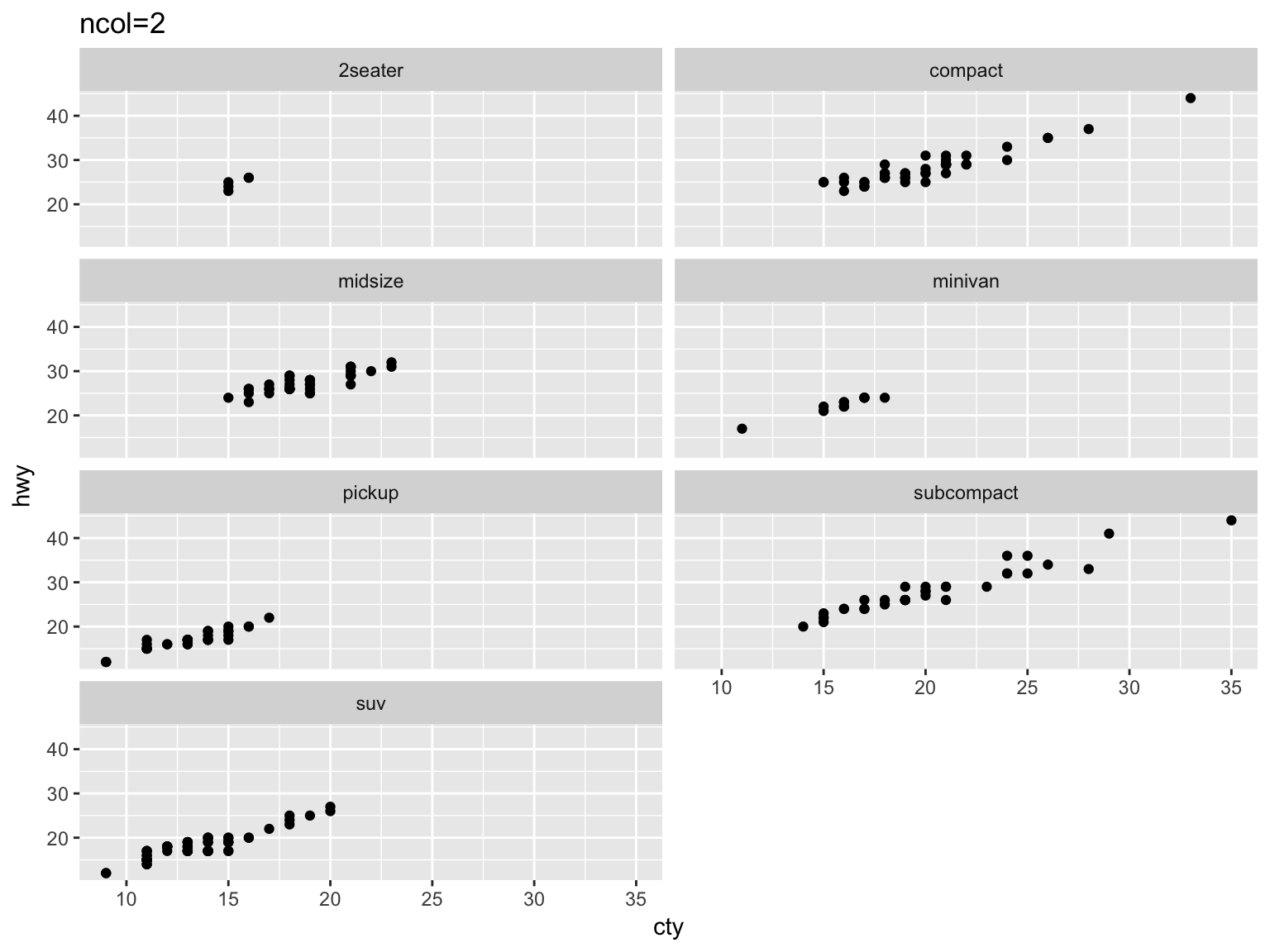## Exercise

Create two graphics showing the relationship between displ and hwy broken down by the categorical variable drv in the dataframe mpg. In one version, use a single row arrangement. In the other, use a single column. Which do you prefer.

``````e = ggplot(data=mpg,aes(x=displ,y=hwy)) + geom_point()
e``````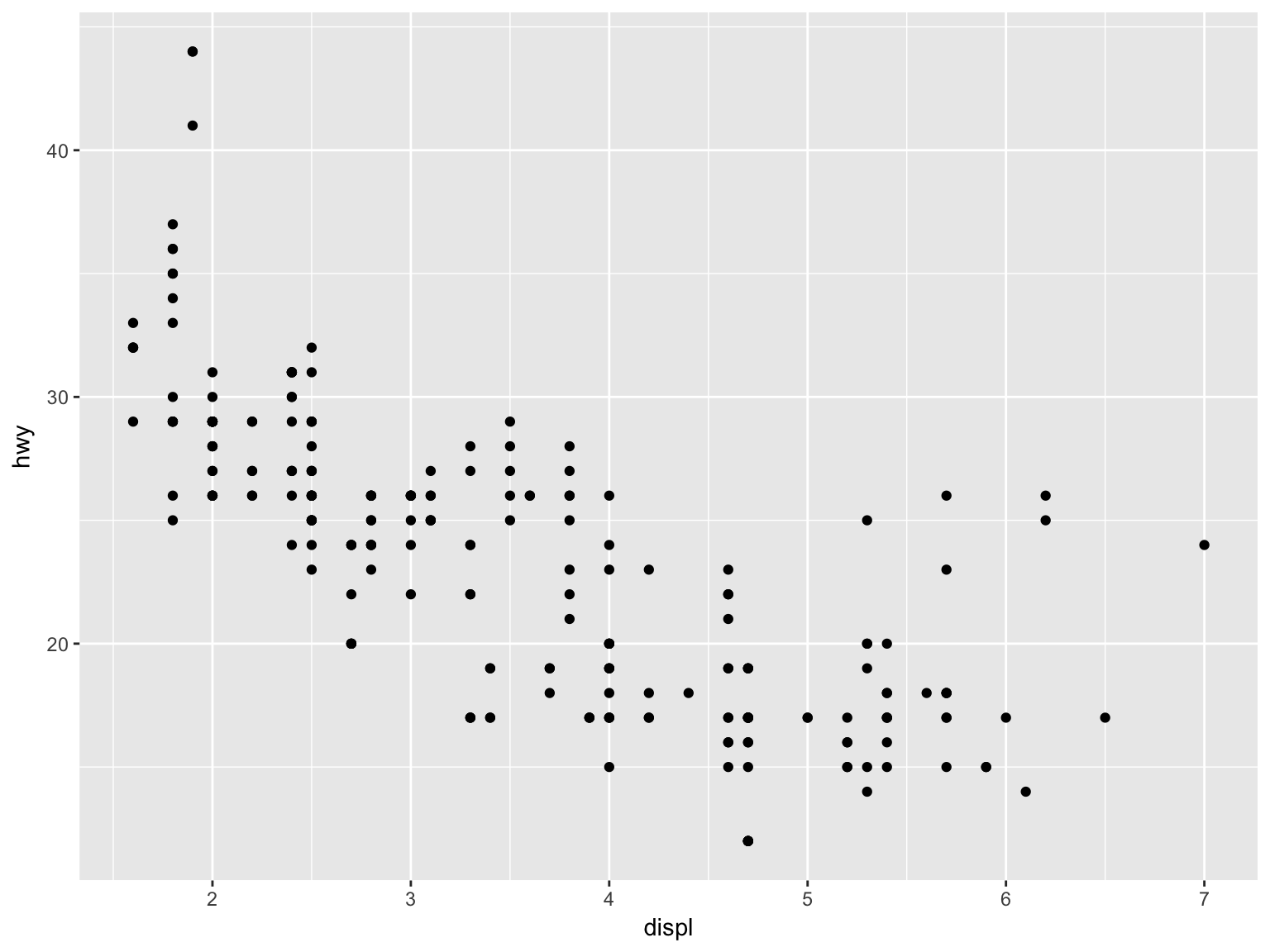``e + facet_wrap(~drv,nrow=1) + ggtitle("nrow=1")``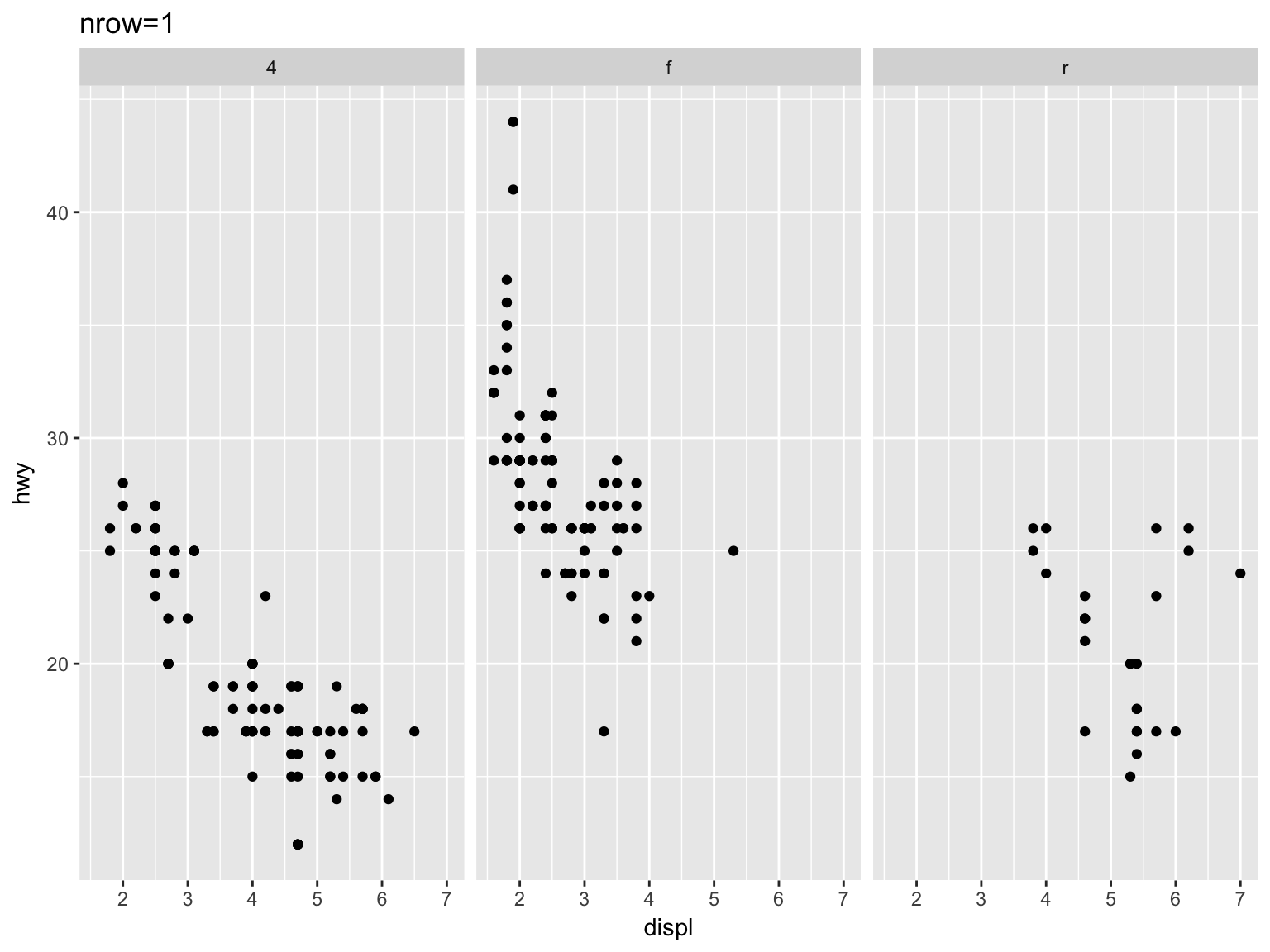``e + facet_wrap(~drv,ncol=1) + ggtitle("ncol=1")``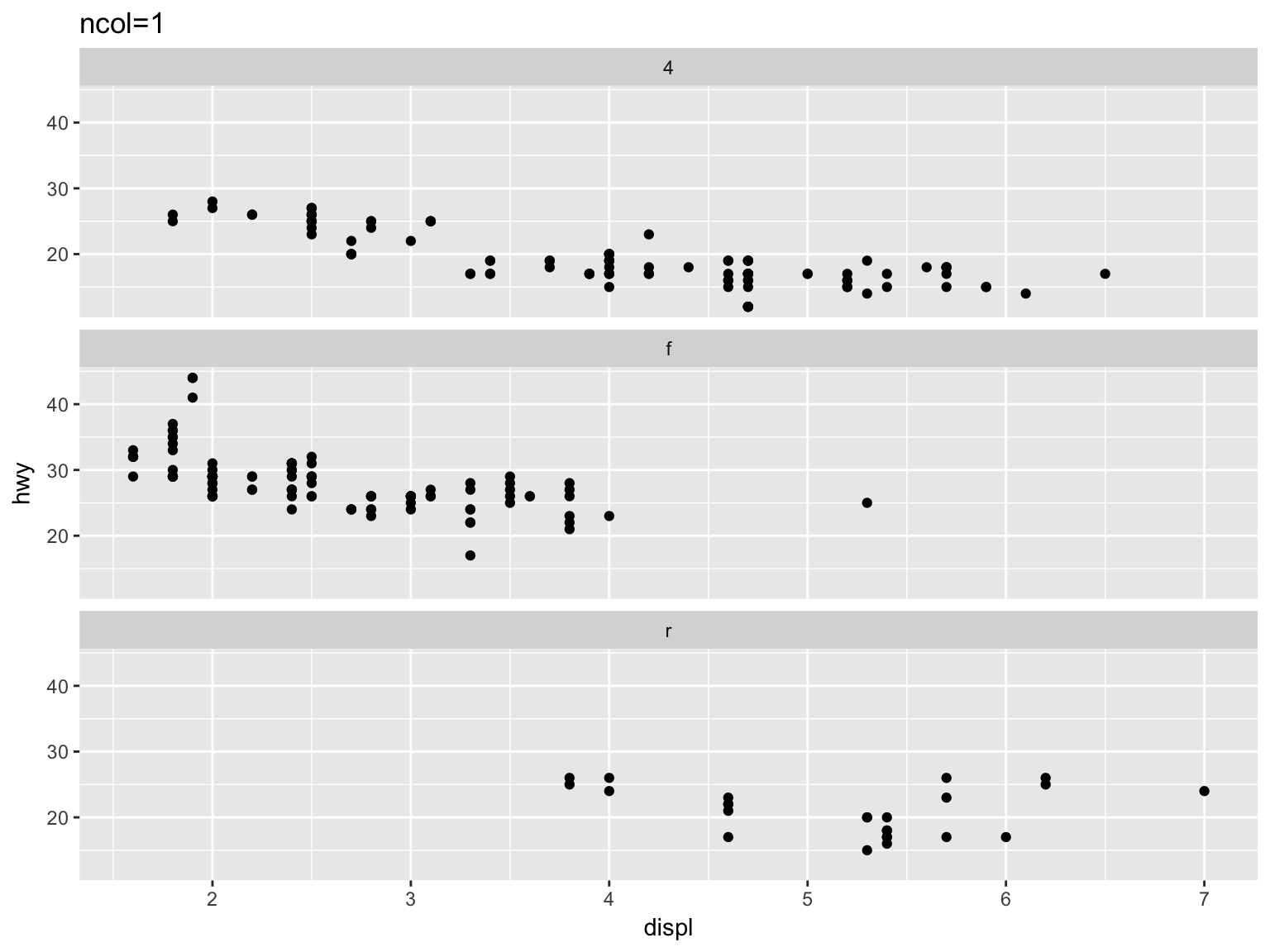## facet_grid

To produce separate graphs for each combination of values of two categorical variables use facet_grid() as a layer. The syntax requires a two-variable formula (two variables separated by a ~). The first variable designates the rows and the second designates the columns.

Example

``````f = ggplot(mpg,aes(x=cty,y=hwy)) + geom_point()
f``````